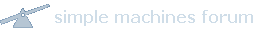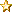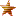#• August 01, 2021, 05:57:30 AM• Welcome, Guest### News:

Welcome to the Bridgetek Community!

Technical Support enquires
@ Bridgetek Support

Please refer to our website for detailed information on all our products - Bridgetek - Bridging Technology

Pages: 

###AuthorTopic: Creating table with rows and columns  (Read 68 times)

#### Geethanjali

• Newbie
•• Posts: 8##### Creating table with rows and columns
« on: July 20, 2021, 09:11:14 AM »

Hi ,

Can you please tell me how to generate rows and columns for Example I need to have 3*3 table also inside this I should be able to insert values.Logged

#### BRT Community

• Sr. Member
•• Posts: 431##### Re: Creating table with rows and columns
« Reply #1 on: July 20, 2021, 04:51:41 PM »

Hello,

There are many ways to create a table. This could be using the built in graphic objects like lines and line strip to make the table. Or you could load an image if you wanted the table to have a particular theme (as you can then add text on top of a bitmap).

You can make it using a series of rectangles. As the rectangles are filled, you can draw two rectangles (the inner one slightly smaller) if you want just an outline.

Note: we used VERTEX2II here as the numbers are clearer (VERTEX2F uses *16 for each value as it uses 1/16th precision by default) but you can use VERTEX2F as it can use coordinates more than 511.
Also, we would recommend to use parameters instead of hard-coded widths etc. as it will make it easier to write the code,

We have a small code snippet of one way to do this too which is from a future appnote and so we can post that too

CLEAR_COLOR_RGB(255, 255, 255)
CLEAR(1, 1, 1)

BEGIN(RECTS)
LINE_WIDTH(16)

// Draw the overall background in black
COLOR_RGB(0, 0, 0)
// Table origin is 0,0 and each cell will be 100 wide by 50 tall
VERTEX2II(0, 0, 0, 0)
VERTEX2II(209, 109, 0, 0)

// Now draw the cells in light blue
COLOR_RGB(85, 170, 255)

// Top-left cell
VERTEX2II(3, 3, 0, 0)   // +3 in X direction, +3 in Y direction (from top-left of table)
VERTEX2II(103, 53, 0, 0)   // +100 in X direction, +50 in Y direction

//Top-Right Cell
VERTEX2II(107, 3, 0, 0)
VERTEX2II(207, 53, 0, 0)

VERTEX2II(3, 57, 0, 0)
VERTEX2II(103, 107, 0, 0)

VERTEX2II(107, 57, 0, 0)
VERTEX2II(207, 107, 0, 0)

END()

COLOR_RGB(0, 0, 0)
CMD_NUMBER(55, 28, 30, OPT_CENTER, 100)
CMD_NUMBER(155, 28, 30, OPT_CENTER, 300)
CMD_NUMBER(55, 82, 30, OPT_CENTER, 200)
CMD_NUMBER(155, 82, 30, OPT_CENTER, 400)

Best Regards, BRT CommunityLogged

#### BRT Community

• Sr. Member
•• Posts: 431##### Re: Creating table with rows and columns
« Reply #2 on: July 22, 2021, 03:57:31 PM »

Hi,

Here is that small example,

Note that this is a basic example without lots of error checking etc. and has only had basic testing so far but but might give you some ideas or be a starting point,

There are lots of ways to draw a table but this one uses just a series of rectangles,

As with the earlier post, it draws a background rectangle the size of the entire table and then draws a series of rectangles inside (one for each cell) with gaps between which become the lines of the table. The functions do the calculations to keep the main code simpler, but are mainly just adding up the dimensions of everything to the left or above the cell currently being drawn so we can work out where to position it.

Using lines or line strip may be easier but using rectangles offers the ability to make the table a bit more attractive. You can add alpha levels etc. to customise the table and cells can be different colours etc.

For really fancy tables, bitmaps may be good.

We'll be using this example along with a better description in an upcoming appnote but hope it helps in the meantime,

Best Regards, BRT Community

Code: [Select]
`#define TABLE_ORIGIN_X 100#define TABLE_ORIGIN_Y 100#define TABLE_CELL_WIDTH 100#define TABLE_CELL_HEIGHT 50#define TABLE_LINE_WIDTH 5// Draws table outline based on the number of columns and rows e.g. (2,2) draws outline for a 2 x 2 tablevoid DrawTableOutline(uint8_t NumColumns, uint8_t NumRows){ uint32_t TABLE_TL_X = 0; uint32_t TABLE_TL_Y = 0; uint32_t TABLE_BR_X = 0; uint32_t TABLE_BR_Y = 0; TABLE_TL_X = TABLE_ORIGIN_X; TABLE_TL_Y = TABLE_ORIGIN_Y; TABLE_BR_X = TABLE_ORIGIN_X  +  ((NumColumns + 1) * TABLE_LINE_WIDTH) + (NumColumns*TABLE_CELL_WIDTH); TABLE_BR_Y = TABLE_ORIGIN_Y  +  ((NumRows + 1) * TABLE_LINE_WIDTH) + (NumRows*TABLE_CELL_HEIGHT); EVE_VERTEX2F((TABLE_TL_X * 16), (TABLE_TL_Y * 16)); EVE_VERTEX2F((TABLE_BR_X * 16), (TABLE_BR_Y * 16));}// Adds a table cell. Note that it is 0-based and so (0,0) is the top left cellvoid DrawTableCell(uint8_t column, uint8_t row){ uint32_t TABLE_CELL_TL_X = 0; uint32_t TABLE_CELL_TL_Y = 0; uint32_t TABLE_CELL_BR_X = 0; uint32_t TABLE_CELL_BR_Y = 0; TABLE_CELL_TL_X = TABLE_ORIGIN_X  +  ((column + 1) * TABLE_LINE_WIDTH) + (column*TABLE_CELL_WIDTH); TABLE_CELL_TL_Y = TABLE_ORIGIN_Y  +  ((row + 1) * TABLE_LINE_WIDTH) + (row*TABLE_CELL_HEIGHT); TABLE_CELL_BR_X = TABLE_ORIGIN_X  +  ((column + 1) * TABLE_LINE_WIDTH) + ((column+1)*TABLE_CELL_WIDTH); TABLE_CELL_BR_Y = TABLE_ORIGIN_Y  +  ((row + 1) * TABLE_LINE_WIDTH) + ((row+1)*TABLE_CELL_HEIGHT); EVE_VERTEX2F((TABLE_CELL_TL_X * 16), (TABLE_CELL_TL_Y * 16)); EVE_VERTEX2F((TABLE_CELL_BR_X * 16), (TABLE_CELL_BR_Y * 16));}// Gets X coordinate of the center of a specified cell (pass in the column number in 0-based format e.g. 1 is the second column)uint32_t GetCellCentreX(uint8_t column){ uint32_t TABLE_CELL_CENTER_X = 0; TABLE_CELL_CENTER_X = TABLE_ORIGIN_X  +  ((column + 1) * TABLE_LINE_WIDTH) + (column*TABLE_CELL_WIDTH) + (TABLE_CELL_WIDTH/2); return TABLE_CELL_CENTER_X;}// Gets Y coordinate of the center of a specified cell (pass in the row number in 0-based format e.g. 1 is the second row)uint32_t GetCellCentreY(uint8_t row){uint32_t TABLE_CELL_CENTER_Y = 0; TABLE_CELL_CENTER_Y = TABLE_ORIGIN_Y  +  ((row + 1) * TABLE_LINE_WIDTH) + (row*TABLE_CELL_HEIGHT) + (TABLE_CELL_HEIGHT/2); return TABLE_CELL_CENTER_Y;}void eve_display(void){ EVE_LIB_BeginCoProList(); EVE_CMD_DLSTART(); EVE_CLEAR_COLOR_RGB(0, 0, 0); EVE_CLEAR(1,1,1); // Begin rectangles with line width 1*16 EVE_BEGIN(EVE_BEGIN_RECTS); EVE_LINE_WIDTH(16); // Draw the table outline in white EVE_COLOR_RGB(255, 255, 255); DrawTableOutline(2, 2); // Draw the cells for a 2 x 2 table // Cell numbers are 0-based EVE_COLOR_RGB(0, 0, 255); DrawTableCell(0, 0); // Draw top-left cell DrawTableCell(0, 1); // Draw bottom-left cell DrawTableCell(1, 0); // Draw top-right cell DrawTableCell(1, 1); // Draw bottom-right cell EVE_END(); // Add numerical values in black text // Use the GetCellCentre functions and enable the EVE_OPT_CENTER EVE_COLOR_RGB(0, 0, 0); EVE_CMD_NUMBER(GetCellCentreX(0), GetCellCentreY(0), 30, EVE_OPT_CENTER, 100); EVE_CMD_NUMBER(GetCellCentreX(0), GetCellCentreY(1), 30, EVE_OPT_CENTER, 200); EVE_CMD_NUMBER(GetCellCentreX(1), GetCellCentreY(0), 30, EVE_OPT_CENTER, 300); EVE_CMD_NUMBER(GetCellCentreX(1), GetCellCentreY(1), 30, EVE_OPT_CENTER, 400); EVE_DISPLAY(); EVE_CMD_SWAP(); EVE_LIB_EndCoProList(); EVE_LIB_AwaitCoProEmpty(); do { } while(1); `Logged
Pages: